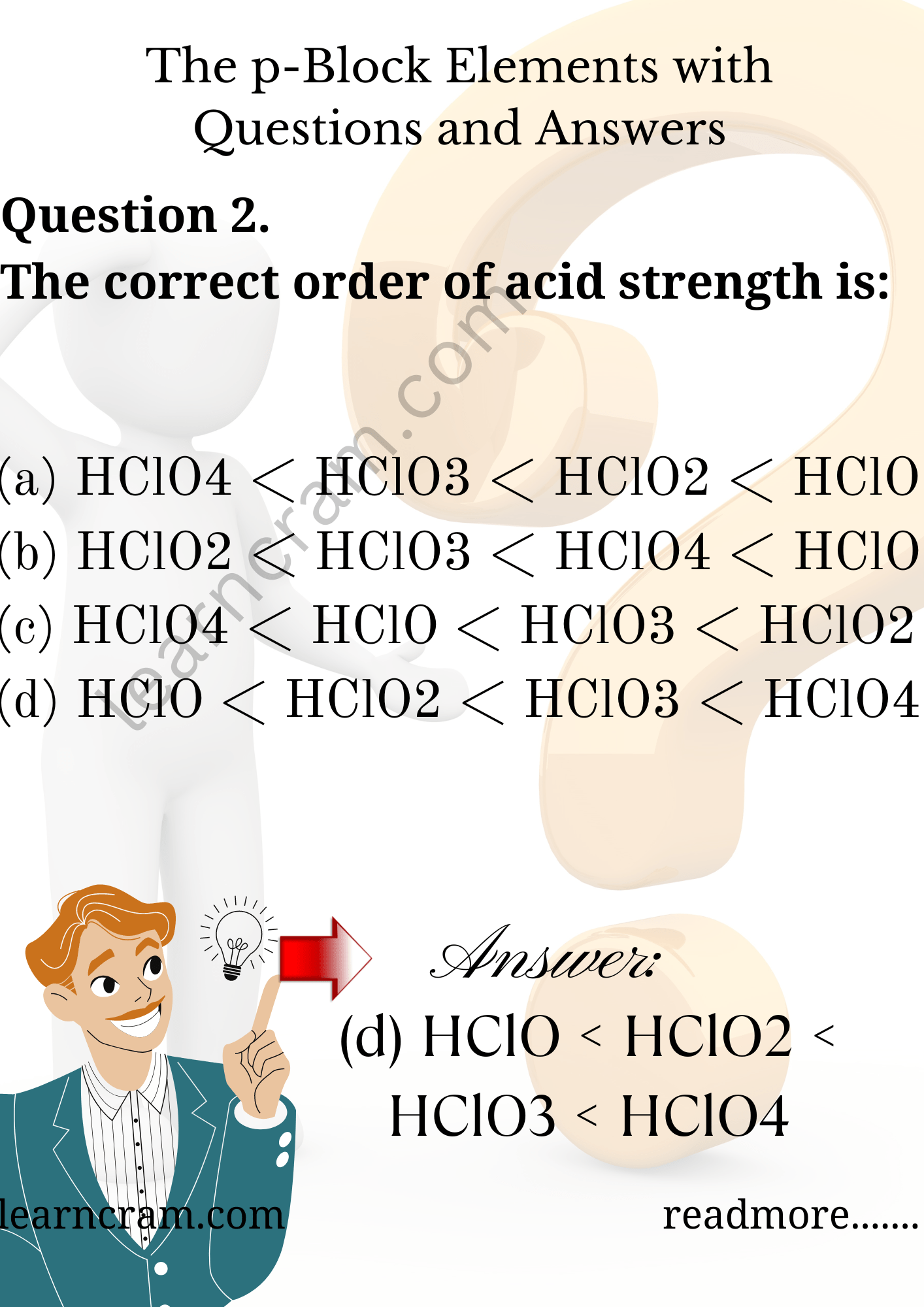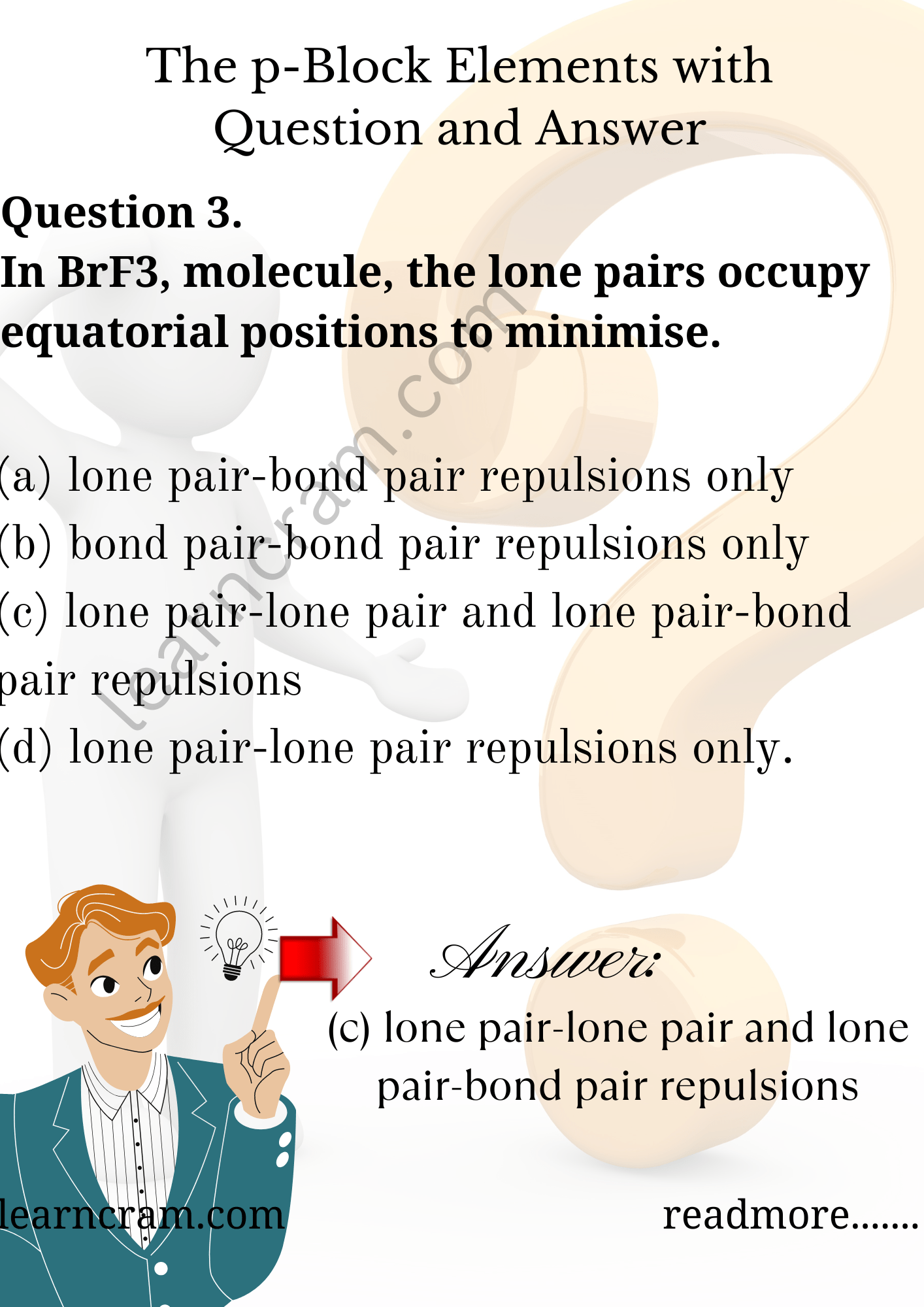# MCQ Questions for Class 12 Chemistry Chapter 7 The p-Block Elements with Answers

We have compiled the NCERT MCQ Questions for Class 12 Chemistry Chapter 7 The p-Block Elements with Answers Pdf free download covering the entire syllabus. Practice MCQ Questions for Class 12 Chemistry with Answers on a daily basis and score well in exams. Refer to the The p-Block Elements Class 12 MCQs Questions with Answers here along with a detailed explanation.

## The p-Block Elements Class 12 MCQs Questions with Answers

Question 1.
The isoelectronic pair is
(a) Cl2O, ICl2
(b) ICl2ClO2
(c) IF2+, I3
(d) ClO2, ClF2+

Question 2.
The correct order of acid strength is:
(a) HClO4 < HClO3 < HClO2 < HClO
(b) HClO2 < HClO3 < HClO4 < HClO
(c) HClO4 < HClO < HClO3 < HClO2
(d) HClO < HClO2 < HClO3 < HClO4

Answer: (d) HClO < HClO2 < HClO3 < HClO4Question 3.
In BrF3, molecule, the lone pairs occupy equatorial positions to minimise.
(a) lone pair-bond pair repulsions only
(b) bond pair-bond pair repulsions only
(c) lone pair-lone pair and lone pair-bond pair repulsions
(d) lone pair-lone pair repulsions only.

Answer: (c) lone pair-lone pair and lone pair-bond pair repulsionsQuestion 4.
The ONO angle is maximum in
(a) NO3
(b) NO2
(c) NO2
(d) NO2+

Question 5.
The correct order of bond angles (smallest first) in H2S, NH3, BF3, SiH4 is
(a) H2S < SiH4 < NH3 < BF3.
(b) NH3 < H2S < SiH4 < BF3
(c) H2S < NH3 < SiH4 < BF3
(d) H2S < NH3 < BF3 < SiH4.

Answer: (c) H2S < NH3 < SiH4 < BF3

Question 6.
Which of the following oxides of nitrogen is solid?
(a) NO2
(b) N2O
(c) N2O3
(d) N2O5

Question 7.
Which one of the following arrangements represents the correct order of electron gain enthalpy (with negative sign) of the given atomic species?
(a) F < Cl < O < S
(b) S < O < Cl < F
(c) O < S < F < Cl
(d) Cl < F < S < 0

Answer: (d) Cl < F < S < 0

Question 8.
Which of the following isomers of phosphorus is thermodynamically most stable?
(a) Red
(b) White
(c) Black
(d) Yellow

Question 9.
Which one of the following contains the maximum number of lone pair of electrons on the central atom?
(a) ClO3
(b) XeF4
(c) SF4
(d) I3

Question 10.
Among the following molecules:
XeO3, XeOF4, XeF6, those containing same number of lone pairs on Xe are:
(a) (i) and (ii) only
(b) (i) and (iii) only
(c) (ii) and (iii) only
(d) (i), (ii) and (iii)

Answer: (d) (i), (ii) and (iii)

Question 11.
Oxidizing power of perhalates decreases in the order
(a) BrO4 > IO4 >ClO4
(b) Cl04 > BrO4 > IO4
(c) IO4 > BrO4 > ClO4
(d) ClO4 > IO4 > BrO4

Answer: (a) BrO4 > IO4 >ClO4

Question 12.
There are no S-S bond in
(a) S2O42-
(b) S2O52
(c) S2O32-
(d) S2O72-

Question 13.
Among the tri halides of Nitrogen, which one is the least basic?
(a) NF3
(b) NCl3
(c) NBr3
(d) Nl3

Question 14.
Which of the following oxides is the most acidic?
(a) N2O5
(b) P2O5
(c) As2O5
(d) Sb2O5

Question 15.
The number of P-O-P bonds in cyclic metaphosphoric acid is
(a) zero
(b) two
(c) three
(d) four

Question 16.
Molecular shapes of SF4, CF4 and XeF4 are:
(a) the same with 2,0, and 1 lone pairs of electrons respectively.
(b) the same with 1,1 and 1 lone pairs of electrons respectively.
(c) different with 0,1 and 2 lone pairs of electrons respectively.
(d) different with 1,0 and 2 lone pairs of electrons respectively.

Answer: (d) different with 1,0 and 2 lone pairs of electrons respectively.

Question 17.
The acid which forms two series of salts is
(a) H3PO4
(b) H3PO3
(c) H3BO3
(d) H3PO2.

Question 18.
Which of the following hydrogen halides is most volatile?
(a) HF
(b) HCl
(c) HBr
(d) HI

Question 19.
The shape of ClO3 is
(a) triangular pyramid
(b) tetrahedral
(c) triangular planer
(d) triangular bipyramid.

Question 20.
The oxidation number of Sulphur in S8, S2F2 and H2S respectively are
(a) 0, +1, -2
(b) +2, + 1 and -2
(c) 0, + 1,+2
(d) -2, + 1, -2.

Question 21.
The true statement for the acids of phosphorus, H3PO2, H3PO3, and H3PO4 is:
(a) The order of their acidity is H3PO4 > H3PO3 > H3PO2.
(b) AH of them are reducing agents.
(c) All of them are tribasic acids.
(d) The geometry of phosphorus is tetrahedral in all the three.

Answer: (a) The order of their acidity is H3PO4 > H3PO3 > H3PO2.

Question 22.
The element which forms oxides in all oxidation states +I to + V is
(a) N
(b) P
(c) As
(d) Sb

Question 23.
For H3PO3 and H3PO4/sub> the correct choice is
(a) H3PO3 is dibasic and reducing.
(b) H3PO3 is dibasic and non-reducing.
(c) H3PO4 is tribasic and reducing.
(d) H3PO3 is tribasic and non-reducing.

Answer: (a) H3PO3 is dibasic and reducing.

Question 24.
The acid having 0-0 bond is
(a) H2S2O3
(b) H2S2O6
(c) H2S2O8
(d) H2S4O6.

Question 25.
Which of the following statement is not true?
(a) Among halide ions, iodide ion is the most powerful reducing agent.
(b) Fluorine is the only halogen which does not show a variable oxidation state.
(c) HOC1 is a stronger acid than HOBr
(d) HF is a stronger acid than HCl.

Answer: (d) HF is a stronger acid than HCl.

Question 26.
Total number of lone pairs of electrons in XtOF4 is
(a) 0
(b) 1
(c) 2
(d) 3

Question 27.
Water is oxidized to oxygen by
(a) ClO2
(b) kMnO4
(c) H2O2
(d) Fluorine
(e) Ozone.

Question 28.
Which of the following are isoelectronic and isostructural?
NO3, CO32-, ClO3, SO3
(a) NO3, CO32-
(b) SO3, NO3
(c) ClO3, CO32-
(d) CO32-, SO3

Question 29.
On boiling phosphorus with KOH solution, product formed is
(a) Potassium sulphate
(b) Phosphorus pentoxide
(c) Phosphorus hydroxide
(d) Phosphine.

Question 30.
Which statement is wrong for NO?
(a) It is anhydride of nitrous acid.
(b) Its dipole moment is 0.22 D.
(c) It forms dimer.
(d) It is paramagnetic.

Answer: (a) It is anhydride of nitrous acid.

Question 31.
Which of the following is not hydrolysed?
(a) ASCl3
(b) PF3
(d) SbCl3
(d) NF3

Question 32.
A pale blue liquid is obtained by equimolar mixture of two gases at -30°C is
(a) N2O
(b) N2O3
(c) N2O4
(d) N2O5

Question 33.
The following acids have been arranged in order of decreasing acid strength. Identify the correct order ClOH (I) BrOH (II) IOH (III)
(a) I > II > III
(b) II > I > III
(c) III > II > I
(d) I > III > II

Answer: (a) I > II > III

Question 34.
When XF4 is treated with water, it forms
(a) Only Xe
(b) Both Xe and XeO3
(c) OnlyXeO3
(d) Both XeO3 and XeOF4.

Answer: (b) Both Xe and XeO3

Question 35.
Which of the following is not formed by Xe?
(a) XeF5
(b) XeF
(c) XeF3
(d) All the three.

Question 36.
The shape and hybridization of some xenon oxy fluorides are given below. Choose the wrong answer.
(a) XeOF2-T shaped-sp³d
(b) XeOF4-square pyramidal-sp³d²
(c) XeO2F2-Trigonal bipyramid-sp³d
(d) XeO3F2-Tetrahedral-sp³.

Question 37.
Which of the following is not a correct-statement?
(a) Solid PCl5 exists as [PBr4]+ Br
(b) Solid PBr5 exists as [PBr4]+ Br
(c) Solid N2O5 exists as NO2+ NO3
(d) Oxides of phosphorus P2O3 and P2O5 exist as monomers.

Answer: (d) Oxides of phosphorus P2O3 and P2O5 exist as monomers.

Question 38.
The noble gas which does not form clathrates is
(a) He
(b) Ar
(c) Kr
(d) Xe.

Question 39.
Which among the following factors is most important in making fluorine the strongest oxidising agent? (AIEEE 2004)
(a) electron affinity
(b) ionisation energy
(c) hydration energy
(d) bond dissociation energy.

Question 40.
Which gas is evolved when PbO2 is treated with cone. HNO3?
(a) NO2
(b) O2
(c) N2
(d) N2O.

Question 41.
Which of the following is the increasing order of enthalpy of vaporization?
(a) NH3, PH3, ASH3
(b) AsH3, PH3, NH3
(c) NH3, ASH3, PH3
(d) PH3, AsH3, NH3

Question 42.
The number of S-S bonds in Sulphur trioxide trimer (S3O9) is
(a) three
(b) two
(c) one
(d) zero PbBr4

Question 43.